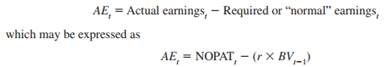### Create an Account

Home / Questions / As discussed in the chapter abnormal earnings AE are where NOPAT is the firms net opera...

# As discussed in the chapter abnormal earnings AE are where NOPAT is the firms net operating profit after taxes r is the cost of equity capital and BVt21 is the book value of equity at time

As discussed in the chapter, abnormal earnings (AE) arewhere NOPAT is the firm’s net operating profit after taxes, r is the cost of equity capital, and BVt21 is the book value of equity at time t 21.

Required:

Solve the following problems:

1. If NOPAT is \$5,000, r 5 15%, and BVt21 is \$50,000, what is AE?

2. If NOPAT is \$25,000, r 5 18%, and BVt21 is \$125,000, what is AE?

3. Assume the firm in requirement 2 can increase NOPAT to \$30,000 by instituting some cost-cutting measures. What is the new AE?

4. Assume the firm in requirement 2 can divest \$25,000 of unproductive capital with NOPAT falling by only \$2,000. What is the new AE?

5. Assume the firm in requirement 2 can add a new division at a cost of \$40,000, which will increase NOPAT by \$7,600 per year. Would adding the new division increase AE?

6. Assume the firm in requirement 1 can add a new division at a cost of \$25,000, which will increase NOPAT by \$3,500 per year. Would adding the new division increase AE?

May 25 2020 View more View LessSubscribe To Get Solution